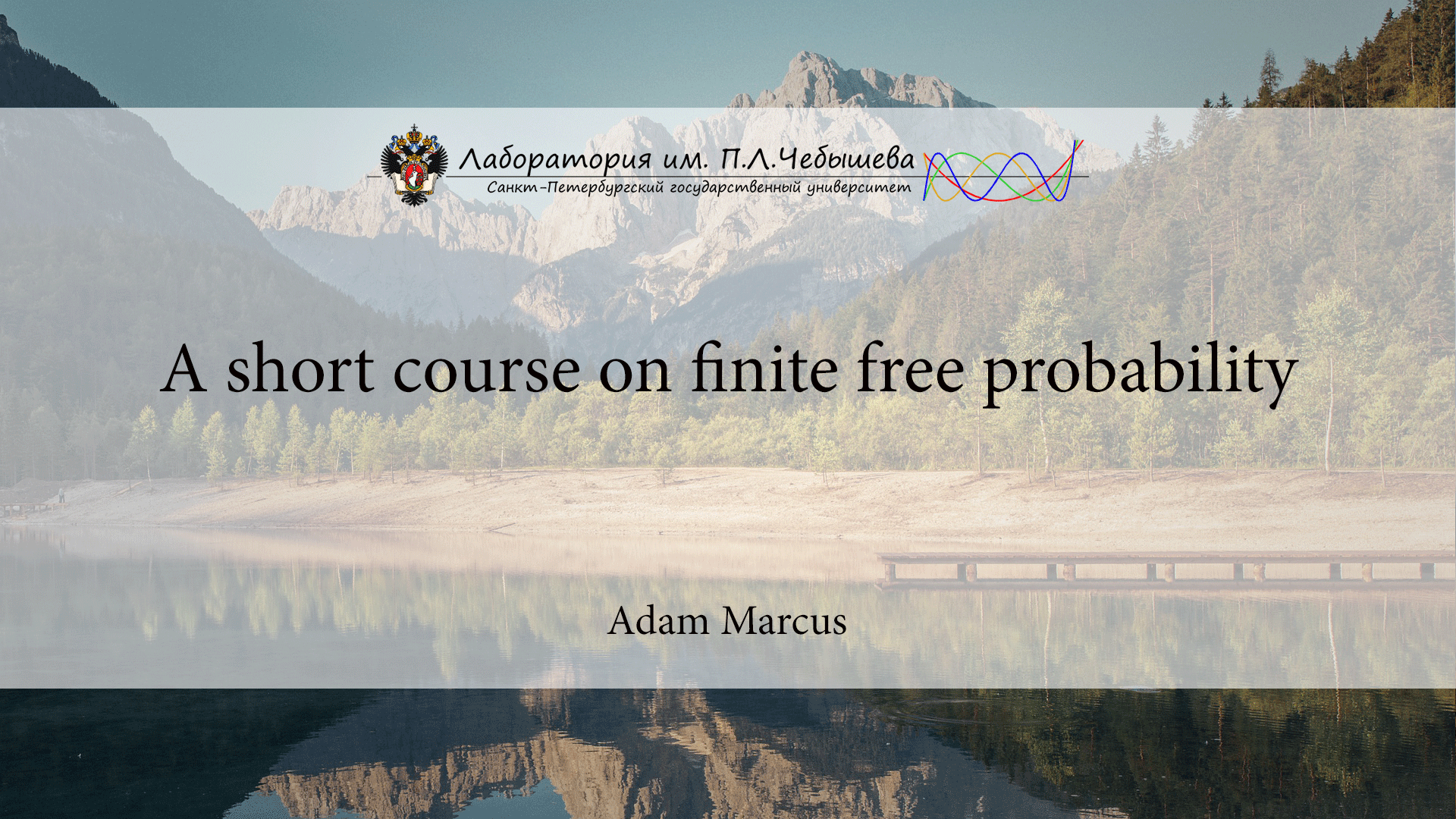# A short course on finite free probability

Курс
Предмет:
Лектор:The theory of free probability has been a useful tool in a number of different areas of mathematics. Most notable, perhaps, is its use in understanding the asymptotic properties of random matrices. One of the limitations of free probability, however, is that the true power of free independence can only be realized in infinite dimensional situations. The goal of this short course will be to introduce a new theory of "finite free probability," which was developed as a way to address these shortcomings.

In the first talk, I will introduce the main concepts of noncommutative probability that I will need and show how they can be adapted to a finite setting. In particular, I hope to show how the finite free additive convolution is a natural extension of the free additive convolution. In the process we will see some of the advantages of having finite structures, including an interesting relationship between classical independence and finite free independence that does not seem to show up in the infinite case.

In the second talk, I will discuss some of the theoretical properties of the finite free additive convolution, including a finite free central limit theorem and finite free entropy. In particular, we will see more of the advantages of dealing with finite structures, including a number of recent and powerful results in the theory of polynomials. This leads to an inequality that suggests that one can actually do better in finite settings than the asymptotic limit would imply.

In the third talk, I will give an application of this new theory to the problem of showing the existence of graphs with particular spectral properties. This will include an argument that we call "the method of interlacing polynomials" which can be seen as an analogue of the probabilistic method from classical probability. In short, this will use more of the recent and powerful results in polynomial theory to show the existence of structures which behave at least as well as the asymptotic limit. I also hope to pose some open questions and suggest possible directions for future research.

3